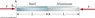# Young's Modulus: 2 bars placed end to end and compressed from both ends

• Angie_Agnes
In summary, a compressive force of 8440 N is applied to the ends of two bars of equal length. The Aluminum bar has a Young's modulus of 7.0*10^10 and the Steel bar has a Young's modulus of 2.0*10^11.f

## Homework Statement

Two bars, one composed of aluminum and one of steel are place end to end as shown in Figure P11.60. The bars are both initially of length L = 24 cm, and both have a square cross section with h = 3.9 cm. If a compressive force of F = 8440 N is applied to each end, find the change in length of the Aluminum=____________ and Steel=___________

Young's Modululs for Aluminum: 7.0*10^10
Young's Modululs for Steel: 2.0*10^11

F/A=y (ΔL/L)

## The Attempt at a Solution

I don't know how to start on this one...

#### Attachments

•11P56.jpeg
10.6 KB · Views: 457
Draw a FBD of the aluminum...how much doest it compress? Draw a FBD of the steel...how much does it compress?

I tried taking the force x2, then using the youngs modulus to try to find ΔL but was getting the wrong answer. Does the compression of the steel affect the side of aluminum that is touching it? do both sides of the bar compress?

I tried taking the force x2,
What do you mean by 'the force x 2'? What is the force on the aluminum bar? What is the force on the steel bar?
then using the youngs modulus to try to find ΔL
yes
.. Does the compression of the steel affect the side of aluminum that is touching it?
The force of the steel on the aluminum affects it
do both sides of the bar compress?
It doesn't matter. If you had a copper bar fixed at one end to a rigid wall and applied a force F to it, it would compress say 1 mm at your end. If the bar was compessed by you pushing it on one end with a force F and your buddy pushing it with a force F at the other end, it would still compress 1 mm, say 0.5mm at your end and 0.5 mm at your buddy's end. Yeahh, Buddy!

Well, the force is applied to both ends, so I took a guess and multiplied the force by 2 and then used that to try to solve for compression of aluminum. Didn't work.

For equilibrium, there must be a force at both ends, equal and opposite. But draw a free body diagram that cuts through the rod and around one end. Use Newton 1. The force in any part of the rod, aluminum or steel, is ____??____ N?

Normal force-? mg?

What I'm having trouble with is how to define the force from the end that would include the other rod- if I'm just looking at the aluminum section, it's got the force pushing on it from one end, 8440 N, and on the other end it has 8440N force pushing in the opposite direction, plus what ever impact it is having on the force of the steel.

Attempt at free body diagram:

#### Attachments

What I'm having trouble with is how to define the force from the end that would include the other rod- if I'm just looking at the aluminum section, it's got the force pushing on it from one end, 8440 N, and on the other end it has 8440N force pushing in the opposite direction, plus what ever impact it is having on the force of the steel.

Attempt at free body diagram:
Your free body diagrams assume the rods rest on a frictionless table, which is OK, and they are almost correct and would be completely correct if you got rid of that plus sign (your '8440 N +' Newtons should just read '8440 N'). By Newton's first law, the force of the steel on the aluminum is 8440 N, and by his 3rd law, the force of the aluminum on the steel is 8440 N. There is no additional "+" force. Even when you look at just half of the aluminum bar, the internal force in the bar must be 8440 N, and that force is caused by the push force of the of the other part of the aluminum rod acting on the first part. Don't add them twice.. If the force on the end of the alum section was 8440 + N, the net force on the bar would be greater than 0, but since the bars are at rest once they compress to their at rest position, the net force must be 0.

I feel like such an idiot. I forgot a key part of the equation when calculating... dividing the force by the area! So that would by why I kept getting the answers wrong on the homework and figured I must be forgetting missing something.
Thanks for your patience and help.

Can anyone please help me out in finding the Young's modulus and Poisson Ratio of standard copper oxide and Ferrous oxide

Can anyone please help me out in finding the Young's modulus and Poisson Ratio of standard copper oxide and Ferrous oxide